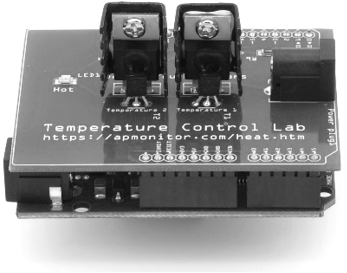## Sensors and Signals for Process ControlAn analog sensors output is typically in the 4-20 mA range. Analog signals are used to transmit sensor readings. A pressure signal of 3-15 psig is typically used to regulate valve position and thereby the fluid flow rate. Valves or pumps are the final control element for many chemical processes.

#### Detect Loss of Signal

When 0 mA is detected, it signals a loss of the sensor. Several causes may include a wire short, break, or loss of power to the sensor. In a pressure line, 0 psig signals a loss of pressure and that the valve cannot be actuated.

#### Gain, Span, Zero

Important terminology is the gain, span, and zero for linear transmitters. The gain is the change in electrical signal divided by the change in process variable. The span is the potential range of process variable that can be measured by that sensor. The zero is the lowest process variable that can be measured by the sensor.

#### Exercise

A flow meter can detect flows up to 200 L/min. A flow of 200 L/min produces a sensor output of 20 mA while a flow of 0 L/min produces a sensor output of 4 mA. Develop an expression for the flow meter and give the transmitter gain, zero, and span.

#### Solution

$$200 \; L/min \to 20 \; mA$$

$$0 \; L/min \to 4 \; mA$$

$$q_m(mA) = \left(\frac{20-4\;mA}{200-0\;L/min}\right)(q - 0 L/min) + 4 \; mA$$

$$Gain = \left(\frac{20-4\;mA}{200-0\;L/min}\right)$$

$$Zero = 0\;L/min$$

$$Span = {200-0\;L/min} = 200 \; L/min$$